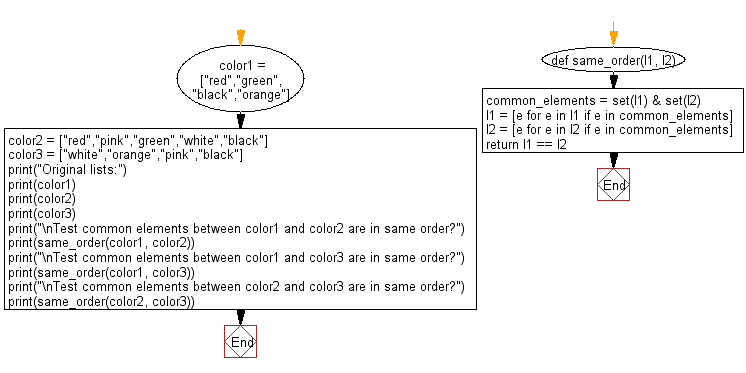﻿ Python: Check common elements between two given list are in same order or not - w3resource# Python: Check common elements between two given list are in same order or not

## Python List: Exercise - 133 with Solution

Write a Python program to check common elements between two given list are in same order or not.

Sample Solution:

Python Code:

``````def same_order(l1, l2):
common_elements = set(l1) & set(l2)
l1 = [e for e in l1 if e in common_elements]
l2 = [e for e in l2 if e in common_elements]
return l1 == l2

color1 = ["red","green","black","orange"]
color2 = ["red","pink","green","white","black"]
color3 = ["white","orange","pink","black"]

print("Original lists:")
print(color1)
print(color2)
print(color3)
print("\nTest common elements between color1 and color2 are in same order?")
print(same_order(color1, color2))
print("\nTest common elements between color1 and color3 are in same order?")
print(same_order(color1, color3))
print("\nTest common elements between color2 and color3 are in same order?")
print(same_order(color2, color3))
```
```

Sample Output:

```Original lists:
['red', 'green', 'black', 'orange']
['red', 'pink', 'green', 'white', 'black']
['white', 'orange', 'pink', 'black']

Test common elements between color1 and color2 are in same order?
True

Test common elements between color1 and color3 are in same order?
False

Test common elements between color2 and color3 are in same order?
False
```

Flowchart:## Visualize Python code execution:

The following tool visualize what the computer is doing step-by-step as it executes the said program:

Python Code Editor:

Have another way to solve this solution? Contribute your code (and comments) through Disqus.

What is the difficulty level of this exercise?

Test your Python skills with w3resource's quiz

﻿

## Python: Tips of the Day

```print(2_000_000)
```2000000